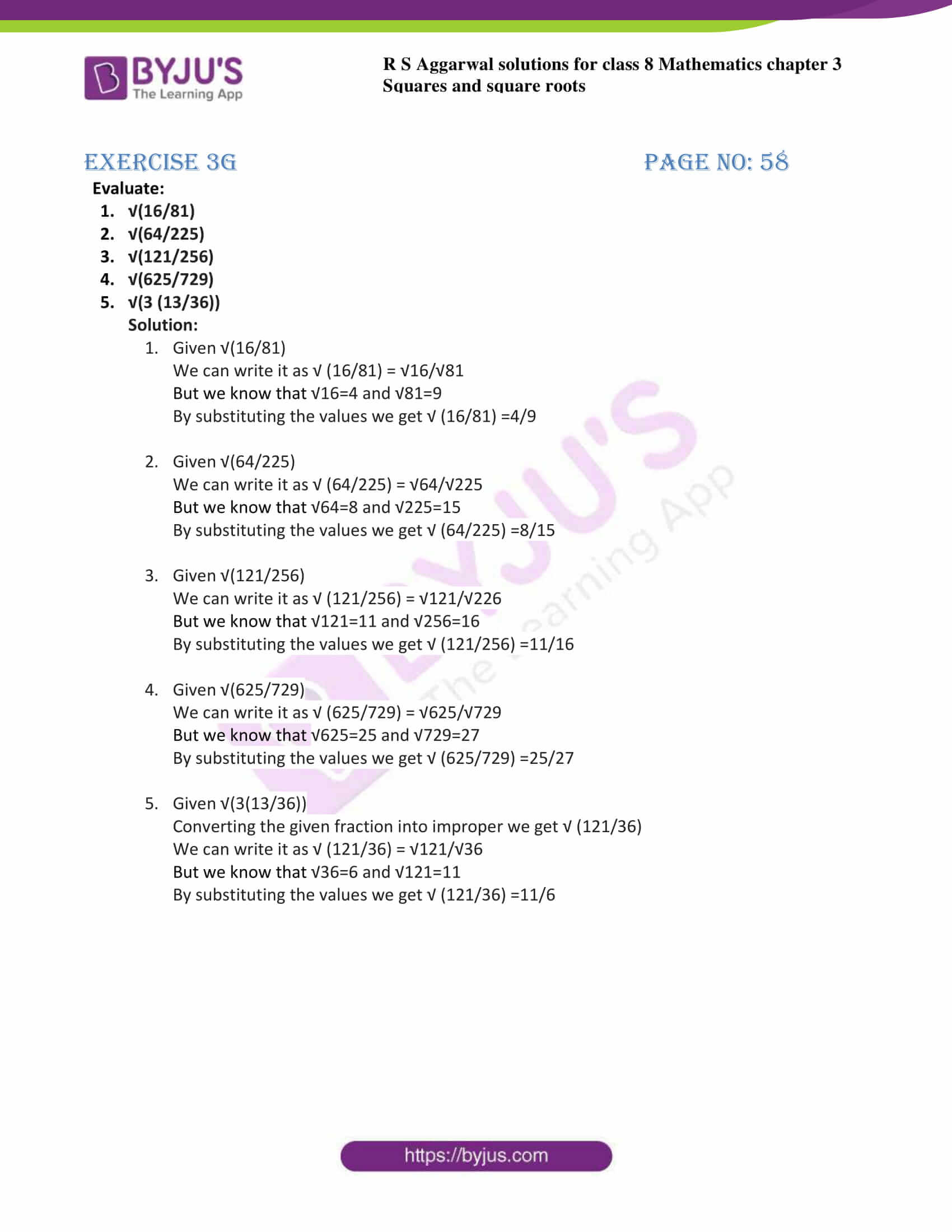# RS Aggarwal Solutions for Class 8 Maths Chapter 3 - Square and Square Roots Exercise 3G

Students can easily download the RS Aggarwal Solutions for the Exercise 3G of Class 8 Maths Chapter 3, Squares and Square Roots from the link accessible here. BYJU’S experts have interpreted the questions from the Exercise 3G in Maths, and this will help students in solving this exercise. The answers are expressed elaborately with steps based on the CBSE syllabus evaluation process. Exercise 3G of Class 8 is about finding square roots of fractions.

, students will be able to grasp the concepts correctly.

## Download PDF of RS Aggarwal Solutions for Class 8 Maths Chapter 3 – Squares and Square Roots – Exercise 3G### Access answers to Maths RS Aggarwal Solutions for Class 8 Chapter 3 – Squares and Square Roots Exercise 3G

1. Evaluate:

1. √(16/81)
2. √(64/225)
3. √(121/256)
4. √(625/729)
5. √(3 (13/36))

Solution:

1. Given √(16/81)We can write it as √ (16/81) = √16/√81But we know that √16=4 and √81=9

By substituting the values we get √ (16/81) =4/9

2. Given √(64/225)We can write it as √ (64/225) = √64/√225But we know that √64=8 and √225=15

By substituting the values we get √ (64/225) =8/15

3. Given √(121/256)We can write it as √ (121/256) = √121/√226But we know that √121=11 and √256=16

By substituting the values we get √ (121/256) =11/16

4. Given √(625/729)We can write it as √ (625/729) = √625/√729But we know that √625=25 and √729=27

By substituting the values we get √ (625/729) =25/27

5. Given √(3(13/36))Converting the given fraction into improper we get √ (121/36)We can write it as √ (121/36) = √121/√36

But we know that √36=6 and √121=11

By substituting the values we get √ (121/36) =11/6

### Access other exercises of RS Aggarwal Solutions for Class 8 Maths Chapter 3 – Squares and Square Roots

Exercise 3A Solutions 6 questions

Exercise 3B Solutions 13 questions

Exercise 3C Solutions 8 questions

Exercise 3D Solutions 18 questions

Exercise 3E Solutions 19 questions

Exercise 3F Solutions 12 questions

Exercise 3H Solutions 19 questions

## RS Aggarwal Solutions for Class 8 Maths Chapter 3 – Squares and Square Roots Exercise 3G

Exercise 3G of RS Aggarwal Solutions for Chapter 3, Squares and Square Roots deals with the basic concepts related to square roots. Exercise 3G contains 10 questions. We can say that this exercise mainly deals with the methods to find square roots of fractions that the students have learned in this chapter. Some of the topics focused prior to exercise 3G include the Square roots of fractions

RS Aggarwal Solutions can help the students in practising and learning each and every concept as it provides solutions to all questions asked in the RS Aggarwal textbook.

#### 1 Comment

1. sanjana sinha

thanks byjus